# Resources tagged with: GeoGebra

Filter by: Content type:
Age range:
Challenge level:

### There are 90 results

Broad Topics > Physical and Digital Manipulatives > GeoGebra### Which Spinners?

##### Age 14 to 18Challenge Level

Can you work out which spinners were used to generate the frequency charts?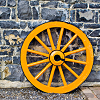### Interactive Spinners

##### Age 11 to 14Challenge Level

This interactivity invites you to make conjectures and explore probabilities of outcomes related to two independent events.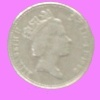### What Does Random Look Like?

##### Age 11 to 14Challenge Level

Engage in a little mathematical detective work to see if you can spot the fakes.### Isosceles Triangles

##### Age 11 to 14Challenge Level

Draw some isosceles triangles with an area of $9$cm$^2$ and a vertex at (20,20). If all the vertices must have whole number coordinates, how many is it possible to draw?### Number Sandwiches

##### Age 7 to 14Challenge Level

Can you arrange the digits 1, 1, 2, 2, 3 and 3 to make a Number Sandwich?### Estimating Angles

##### Age 7 to 14Challenge Level

How good are you at estimating angles?### Factor Lines

##### Age 7 to 14Challenge Level

Arrange the four number cards on the grid, according to the rules, to make a diagonal, vertical or horizontal line.### Teddy Town

##### Age 5 to 14Challenge Level

There are nine teddies in Teddy Town - three red, three blue and three yellow. There are also nine houses, three of each colour. Can you put them on the map of Teddy Town according to the rules?### Colour in the Square

##### Age 7 to 16Challenge Level

Can you put the 25 coloured tiles into the 5 x 5 square so that no column, no row and no diagonal line have tiles of the same colour in them?### Right Angles

##### Age 11 to 14Challenge Level

Can you make a right-angled triangle on this peg-board by joining up three points round the edge?### Points in Pairs

##### Age 14 to 16Challenge Level

Move the point P to see how P' moves. Then use your insights to calculate a missing length.### Solving Together - Estimating Angles

##### Age 11 to 14

Week 2
How well can you estimate angles? Playing this game could improve your skills.### Where Is the Dot?

##### Age 14 to 16Challenge Level

A dot starts at the point (1,0) and turns anticlockwise. Can you estimate the height of the dot after it has turned through 45 degrees? Can you calculate its height?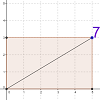### Exploring Diagonals

##### Age 11 to 16

Move the corner of the rectangle. Can you work out what the purple number represents?### The Farmers' Field Boundary

##### Age 11 to 14Challenge Level

The farmers want to redraw their field boundary but keep the area the same. Can you advise them?### Round and Round and Round

##### Age 11 to 14Challenge Level

Where will the point stop after it has turned through 30 000 degrees? I took out my calculator and typed 30 000 ÷ 360. How did this help?### Tilting Triangles

##### Age 14 to 16Challenge Level

A right-angled isosceles triangle is rotated about the centre point of a square. What can you say about the area of the part of the square covered by the triangle as it rotates?### Squirty

##### Age 14 to 16Challenge Level

Using a ruler, pencil and compasses only, it is possible to construct a square inside any triangle so that all four vertices touch the sides of the triangle.### Beelines

##### Age 14 to 16Challenge Level

Is there a relationship between the coordinates of the endpoints of a line and the number of grid squares it crosses?### Exploring Cubic Functions

##### Age 14 to 18Challenge Level

Quadratic graphs are very familiar, but what patterns can you explore with cubics?### Subtended Angles

##### Age 11 to 14Challenge Level

What is the relationship between the angle at the centre and the angles at the circumference, for angles which stand on the same arc? Can you prove it?### Magic Potting Sheds

##### Age 11 to 14Challenge Level

Mr McGregor has a magic potting shed. Overnight, the number of plants in it doubles. He'd like to put the same number of plants in each of three gardens, planting one garden each day. Can he do it?### Shear Magic

##### Age 11 to 14Challenge Level

Explore the area of families of parallelograms and triangles. Can you find rules to work out the areas?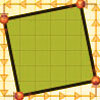### At Right Angles

##### Age 14 to 16Challenge Level

Can you decide whether two lines are perpendicular or not? Can you do this without drawing them?### Triangle in a Triangle

##### Age 14 to 16Challenge Level

Can you work out the fraction of the original triangle that is covered by the inner triangle?### More Twisting and Turning

##### Age 11 to 16Challenge Level

It would be nice to have a strategy for disentangling any tangled ropes...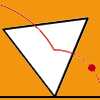### Speeding Up, Slowing Down

##### Age 11 to 14Challenge Level

Experiment with the interactivity of "rolling" regular polygons, and explore how the different positions of the red dot affects its speed at each stage.### Triangles in Circles

##### Age 11 to 14Challenge Level

Can you find triangles on a 9-point circle? Can you work out their angles?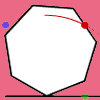### Up and Across

##### Age 11 to 14Challenge Level

Experiment with the interactivity of "rolling" regular polygons, and explore how the different positions of the dot affects its vertical and horizontal movement at each stage.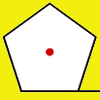### How Far Does it Move?

##### Age 11 to 14Challenge Level

Experiment with the interactivity of "rolling" regular polygons, and explore how the different positions of the red dot affects the distance it travels at each stage.### Rolling Around

##### Age 11 to 14Challenge Level

A circle rolls around the outside edge of a square so that its circumference always touches the edge of the square. Can you describe the locus of the centre of the circle?### Pythagoras Proofs

##### Age 14 to 16Challenge Level

Can you make sense of these three proofs of Pythagoras' Theorem?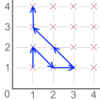### Areas from Vectors

##### Age 11 to 16

Use the applet to explore the area of a parallelogram and how it relates to vectors.### Coordinates of Corners

##### Age 11 to 16

Use the applet to make some squares. What patterns do you notice in the coordinates?### Napoleon's Theorem

##### Age 14 to 18Challenge Level

Triangle ABC has equilateral triangles drawn on its edges. Points P, Q and R are the centres of the equilateral triangles. What can you prove about the triangle PQR?### Just Rolling Round

##### Age 14 to 16Challenge Level

P is a point on the circumference of a circle radius r which rolls, without slipping, inside a circle of radius 2r. What is the locus of P?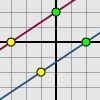### Translating Lines

##### Age 11 to 14Challenge Level

Investigate what happens to the equation of different lines when you translate them. Try to predict what will happen. Explain your findings.### Square Coordinates

##### Age 11 to 14Challenge Level

A tilted square is a square with no horizontal sides. Can you devise a general instruction for the construction of a square when you are given just one of its sides?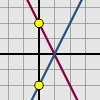### Reflecting Lines

##### Age 11 to 14Challenge Level

Investigate what happens to the equations of different lines when you reflect them in one of the axes. Try to predict what will happen. Explain your findings.##### Age 14 to 16Challenge Level

The points P, Q, R and S are the midpoints of the edges of a non-convex quadrilateral.What do you notice about the quadrilateral PQRS and its area?### Squaring the Circle and Circling the Square

##### Age 14 to 16Challenge Level

If you continue the pattern, can you predict what each of the following areas will be? Try to explain your prediction.### Guesswork

##### Age 14 to 16Challenge Level

Ask a friend to choose a number between 1 and 63. By identifying which of the six cards contains the number they are thinking of it is easy to tell them what the number is.### Reflecting Squarely

##### Age 11 to 14Challenge Level

In how many ways can you fit all three pieces together to make shapes with line symmetry?### Tessellation Interactivity

##### Age 7 to 16Challenge Level

An environment that enables you to investigate tessellations of regular polygons##### Age 14 to 16Challenge Level

Make five different quadrilaterals on a nine-point pegboard, without using the centre peg. Work out the angles in each quadrilateral you make. Now, what other relationships you can see?### More Magic Potting Sheds

##### Age 11 to 14Challenge Level

The number of plants in Mr McGregor's magic potting shed increases overnight. He'd like to put the same number of plants in each of his gardens, planting one garden each day. How can he do it?### Farey Sequences

##### Age 11 to 14Challenge Level

There are lots of ideas to explore in these sequences of ordered fractions.### Partitioning Revisited

##### Age 11 to 14Challenge Level

We can show that (x + 1)² = x² + 2x + 1 by considering the area of an (x + 1) by (x + 1) square. Show in a similar way that (x + 2)² = x² + 4x + 4### Sine and Cosine for Connected Angles

##### Age 14 to 16Challenge Level

The length AM can be calculated using trigonometry in two different ways. Create this pair of equivalent calculations for different peg boards, notice a general result, and account for it.### Mapping the Territory

##### Age 14 to 18Challenge Level

Can you devise a system for making sense of complex multiplication?